Trigonometric FunctionsCustom SearchTRIGONOMETRIC FUNCTIONS If we are given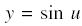we may state that, from the general formula,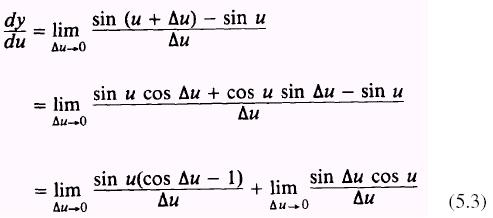SinceandThen by substituting equations (5.4) and (5.5) into equation (5.3),Now we are interested in finding the derivative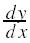of the function sin u, so we apply the chain ruleFrom the chain rule and equation (5.6), we findIn other words, to find the derivative of the sine of a function, we use the cosine of the function times the derivative of the function. By a similar process we find the derivative of the cosine func�tion to be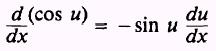The derivatives of the other trigonometric functions may be found by expressing them in terms of the sine and cosine. That is,and by substituting sin u for u, cos u for v, and du for dx in the expression of the quotient theorem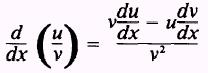we have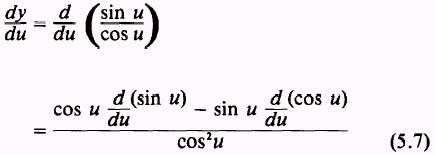Taking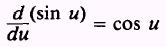and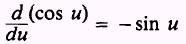and substituting into equation (5.7), we find thatNow using the chain rule and equation (5.8), we find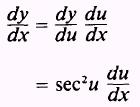By stating the other trigonometric functions in terms of the sine and cosine and using similar processes, we may find the following derivatives: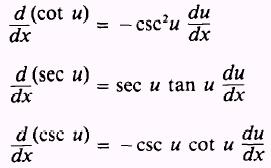EXAMPLE: Find the derivative of the functionSOLUTION.EXAMPLE. Find the derivative of the functionSOLUTION: Use the power theorem to find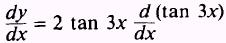Then findand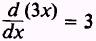Combining all of these, we find thatPRACTICE PROBLEMS: Find the derivative of the following:ANSWERS:Privacy Statement - Copyright Information. - Contact UsIntegrated Publishing, Inc.